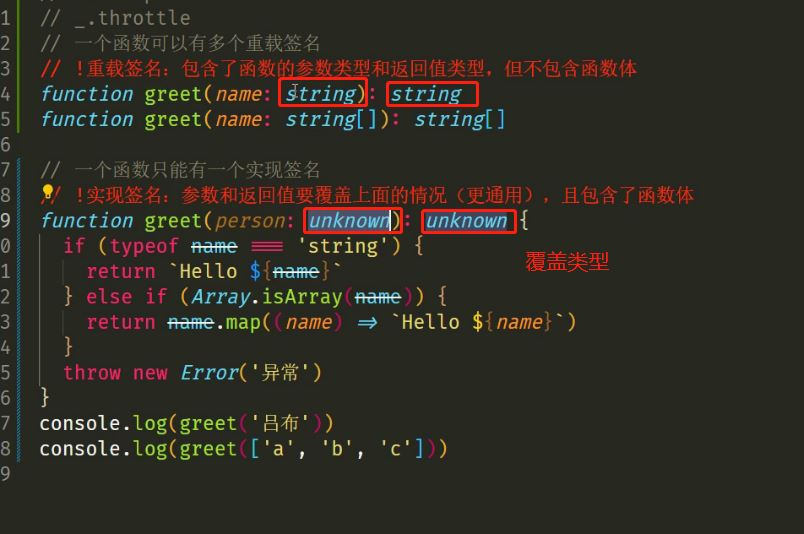# TypeScript基本類型之typeof和keyof詳解

• 安裝命令：`npm i -g typescript` 或者 `yarn global add typescript`
• 驗證是否安裝成功：tsc –v（查看 TypeScript 的版本）。

## 編譯並運行 TS 代碼

1. 創建 `hello.ts` 文件（註意：TS 文件的後綴名為 `.ts`）。
2. 將 TS 編譯為 JS：在終端中輸入命令，`tsc hello.ts`（此時，在同級目錄中會出現一個同名的 JS 文件）。
3. 執行 JS 代碼：在終端中輸入命令，`node hello.js`
4. 說明：所有合法的 JS 代碼都是 TS 代碼，有 JS 基礎隻需要學習 TS 的類型即可。
5. 註意：由 TS 編譯生成的 JS 文件，代碼中就沒有類型信息瞭。

創建 `.`TS文件hello 通過 tsc hello.ts 命令會生成同名js文件 通過node hello`.js運行js文件`

## TypeScript基礎

```let name:string='張三'
let age:number=18

:number 和 :string 就是類型註解```

TS常用基礎類型細分為兩類 分別是JS已有類型和TS新增類型

js已有類型  number ,string boolean null undefined  symbol 和 bigint

Javascript自es6後一共有 7 種原始類型 和 1 種 引用類型，如下：

TS新增類型有

a,聯合類型 ,自定義類型(類型別名type..), 接口 元組 字面量類型 枚舉 void ang等

b,註意：TS 中的原始類型和 JS 中寫法一致；TS 中的對象類型在 JS 類型基礎上更加細化，每個具體的對象（比如數組、對象、函數）都有自己的類型語法。

## 數組類型   []

arr是數組類型,數組裡面裝的是number類型

```const arr=[1,2,3]

// 定義

const arr:number[]=[1,2,3]
arr.push(8)```

## 聯合類型  |

`const temp :number| string =8`

```const arr:number| string[]=[1,'1'] // 不行報錯 優先級問題
// arr是 number類型或者string組成的數組類型

const arr:(number| string)[]=[1,'1']```如果打印 結果   ts文件報錯可以加 export{}來解決

```const arr:Array<number>=[1,2,3]

const aee:Array<number|string>=[1,'1']```

聯合類型的應用場景

```let timer =null
timer =setInterval(()=>{},2000)```

```let timer = number= -1
timer=setInterval(()=>{},2000)```

```let timer= null |number =null
timer=setInterval(()=>{})```

## 類型別名

```let arr1: (number | string)[] = [1, 'a', 3, 'b']
let arr2: (number | string)[] = ['x', 'y', 6, 7]

type CustomArray = (number | string)[]

let arr1: CustomArray = [1, 'a', 3, 'b']
let arr2: CustomArray = ['x', 'y', 6, 7]```

a，使用 `type` 關鍵字來創建自定義類型。

b，類型別名（比如，此處的 CustomArray）可以是任意合法的變量名稱。

c，推薦使用大寫字母開頭。

d，創建類型別名後，直接使用該類型別名作為變量的類型註解即可。

## 函數類型```// 解釋：可以通過類似箭頭函數形式的語法來為函數添加類型，註意這種形式隻適用於函數表達式。
type AddFn = (num1: number, num2: number) => number

return num1 + num2
}```

## void類型

```function greet(name: string): void {
console.log('Hello', name)
// return undefined // 默認有這麼一句
}```

```const add = (): undefined => {
return undefined
}```

## 可選參數 ?

```function mySplice(test:number , start?: number,end?:number){
mySplice(1)
mySplice()
mySplice(1,2)
可選參數不能出現在必選參數前面  必須在必選函數後面  一般配合類型註解使用
}```

## 參數默認值=## 對象類型 :object

`const obj:object = {}`

```const person:{name:string;age:number} ={
name:"zhansan",
age:18
}```## interface

interface +接口名用來描述對象類型type 和interface的區別

都可以用來描述對象 或者函數  都允許進行拓展 ,語法不同

1.   interface的接口拓展通過extends   type的接口拓展通過&

2.  type 加=  interface不加=

3.type可以描述任意類型  interface隻能用來描述對象或函數

4.相同的interface聲明能夠合並  相同的type聲明會報錯  (命名名字)

## 元組類型

// 可以給元組中的元素起別名## 類型推論

```let str1 = 'Hello TS'   //string類型
const str2 = 'Hello TS'   // 字符串字面量類型```

```type Direction = 'up' | 'down' | 'left' | 'right'
function changeDirection(direction: Direction) {
console.log(direction)
}
changeDirection('up') // 調用函數時，會有類型提示```

```// 創建枚舉
enum Direction {
Up,
Down,
Left,
Right,
}

// 可以當做類型使用枚舉
function changeDirection(direction: Direction) {
console.log(direction)
}

// 也可以當做值使用枚舉
// 調用函數時，需要傳入：枚舉 Direction 成員的任意一個，類似於 JS 中的對象，直接通過點（.）語法 訪問枚舉的成員
changeDirection(Direction.Up)```

數字枚舉

```enum Direction {
Up = 2,
Down = 4,
Left = 8,
Right = 16,
}
console.log(Direction['Up']) // 2
// 也可以反向操作
console.log(Direction) // Up```

• 枚舉類型比較特殊，不僅僅可以用作類型，還可以當做值使用，因為枚舉成員都是有值的。
• 也就是說，其他的類型會在編譯為 JS 代碼時自動移除，但是，枚舉類型會被編譯為 JS 代碼
• 說明：枚舉與前面講到的字面量類型 + 聯合類型組合的功能類似，都用來表示一組明確的可選值列表。
• 推薦：字面量類型 + 聯合類型組合的方式，因為相比枚舉，這種方式更加直觀、簡潔、高效。

• 定義：枚舉成員的值是字符串稱為字符串枚舉。
• 註意：字符串枚舉沒有自增長行為，因此，字符串枚舉的每個成員必須有初始值
```enum Gender {
女,
男,
}
type User = {
name: string
age: number
// gender: '男' | '女' // 但後臺需要 0 和 1
gender: Gender
}

const user: User = {
name: 'ifer',
age: 18,
gender: Gender.男,
}```

## 類型斷言  as 或者 <>泛型## typeof

js中判斷某個數據的類型

```let a = 8
console.log(typeof a)// 'number'```

ts中的typeof是 根據已有的值 來獲取值的類型  來簡化代碼的書寫## keyof

```// 接口
interface Person {
name: string
age: number
}
type K1 = keyof Person // "name" | "age"
type K2 = keyof Person[] // "length" | "toString" | "pop" | "push" | "concat" | "join"

// 錯誤寫法
const a =keyof Person```

1.先獲取到對象的類型 通過typeof  2.獲取對象類型所組成的聯合類型

```const a ={name:"張三",age:18}
type keyofa =keyof typeof a```

## any和unknow的區別

unknow 是更加安全的any類型

## 函數重載

函數簽名

```函數類型的第一種情況
function greet(name:string) :string

function greet(name:string[]):string[]```

```function gerrt(name:unknown) :unknow{
if(Array.isArray(name)){
return name.map((item)=>`Hello\${item}`)
}
return `Hello\${name}`
}
greet('xxx')  // 後跟字符串方法
greet(['1','2']) //數組方法```